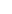RSI is one of the most popular indicators and is designed to indicate "overbought" and "oversold" situations. RSI’s values range between "0" representing the most "oversold" status and "100" representing the most "overbought" status of the coin. RSI is preset to calculate 14 periods. As we reduce the periods the indicator calculates, the more volatile it becomes. Usually, the shorter the trading intervals we want to make, the fewer periods of time we use. The mathematical formula for calculating the RSI is as follows:

RSI = 100 - (100/1 + RS) where:
RS = (sum of the upward closing values / n) / (sum of the downward closing values / n)
n = The number of periods

As we can see, the indicator basically compares the upward and downward dynamics of a coin, giving us an insight into the pressure (buying or selling) that the coin takes. We can distinguish three levels of significance in the RSI’s graph: the overbought level when RSI exceeds the value of 70, the oversold level when RSI’s value drops below 30, and the centerline (50). If the overbought limit is exceeded we receive a sell signal, if the oversold level is exceeded we get a buy signal, and depending on the direction that follows when it breaks the centerline it confirms an upward or a downward trend.

When the price of a coin reaches new highs (or lows) and the RSI does not record new higher (or lower) values, there is an indication of reversal of the trend.to signal pageRelative Strength Index - RSI ❺❺❺ - average rating 5 from 5 (based on 201 user reviews)